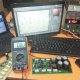Breaking News
Home / Electronics / Voltage controller circuit with ampere using IRF540N? electronics

# Voltage controller circuit with ampere using IRF540N? electronics

## Voltage controller circuit with ampere using IRF540N:

This is a Voltage controller circuit with ampere using IRF540N. IRF540N. This transistor can take 33Amperes and  100Voltage. 0.040 Ohm, N-channel Power MOSFET. This is only DC voltage Regulator. we can control 0 voltage to 100 voltage. But here in this video, I use 35 voltage.

This is the circuit Diagram. easily we can make this circuit diagram.

We make this circuit diagram and test. so, Let’s go to watch the video